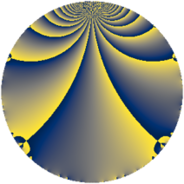Properties

 Label 1089.3.iLevel $1089$ Weight $3$ Character orbit 1089.i Rep. character $\chi_{1089}(122,\cdot)$ Character field $\Q(\zeta_{6})$ Dimension $418$ Sturm bound $396$

Related objects

Defining parameters

 Level: $$N$$ $$=$$ $$1089 = 3^{2} \cdot 11^{2}$$ Weight: $$k$$ $$=$$ $$3$$ Character orbit: $$[\chi]$$ $$=$$ 1089.i (of order $$6$$ and degree $$2$$) Character conductor: $$\operatorname{cond}(\chi)$$ $$=$$ $$9$$ Character field: $$\Q(\zeta_{6})$$ Sturm bound: $$396$$

Dimensions

The following table gives the dimensions of various subspaces of $$M_{3}(1089, [\chi])$$.

Total New Old
Modular forms 552 454 98
Cusp forms 504 418 86
Eisenstein series 48 36 12

Trace form

 $$418 q + 3 q^{2} + 3 q^{3} + 401 q^{4} - 6 q^{5} + 13 q^{6} + 25 q^{9} + O(q^{10})$$ $$418 q + 3 q^{2} + 3 q^{3} + 401 q^{4} - 6 q^{5} + 13 q^{6} + 25 q^{9} + 12 q^{10} + 44 q^{12} + 6 q^{13} + 12 q^{14} + 38 q^{15} - 731 q^{16} - 4 q^{18} + 6 q^{19} - 120 q^{20} + 10 q^{21} - 126 q^{23} - 69 q^{24} + 863 q^{25} + 12 q^{27} - 36 q^{28} + 138 q^{29} - 20 q^{30} - 63 q^{32} + 5 q^{34} + 35 q^{36} + 24 q^{37} + 33 q^{38} - 8 q^{39} + 30 q^{40} + 237 q^{41} - 198 q^{42} + 3 q^{43} - 332 q^{45} + 120 q^{46} + 210 q^{47} - 277 q^{48} - 1073 q^{49} + 483 q^{50} - 35 q^{51} - 66 q^{52} + 193 q^{54} - 72 q^{56} - 117 q^{57} - 12 q^{58} + 345 q^{59} + 592 q^{60} - 54 q^{61} + 56 q^{63} - 2614 q^{64} + 282 q^{65} + 29 q^{67} - 855 q^{68} - 286 q^{69} + 148 q^{70} - 171 q^{72} + 66 q^{73} - 156 q^{74} + 37 q^{75} - 33 q^{76} + 176 q^{78} + 72 q^{79} - 455 q^{81} + 258 q^{82} - 708 q^{83} + 656 q^{84} - 42 q^{85} - 393 q^{86} - 474 q^{87} + 1070 q^{90} - 36 q^{91} - 1032 q^{92} - 738 q^{93} - 18 q^{94} + 78 q^{95} - 484 q^{96} + 39 q^{97} + O(q^{100})$$

Decomposition of $$S_{3}^{\mathrm{new}}(1089, [\chi])$$ into newform subspaces

The newforms in this space have not yet been added to the LMFDB.

Decomposition of $$S_{3}^{\mathrm{old}}(1089, [\chi])$$ into lower level spaces

$$S_{3}^{\mathrm{old}}(1089, [\chi]) \cong$$ $$S_{3}^{\mathrm{new}}(9, [\chi])$$$$^{\oplus 3}$$$$\oplus$$$$S_{3}^{\mathrm{new}}(99, [\chi])$$$$^{\oplus 2}$$